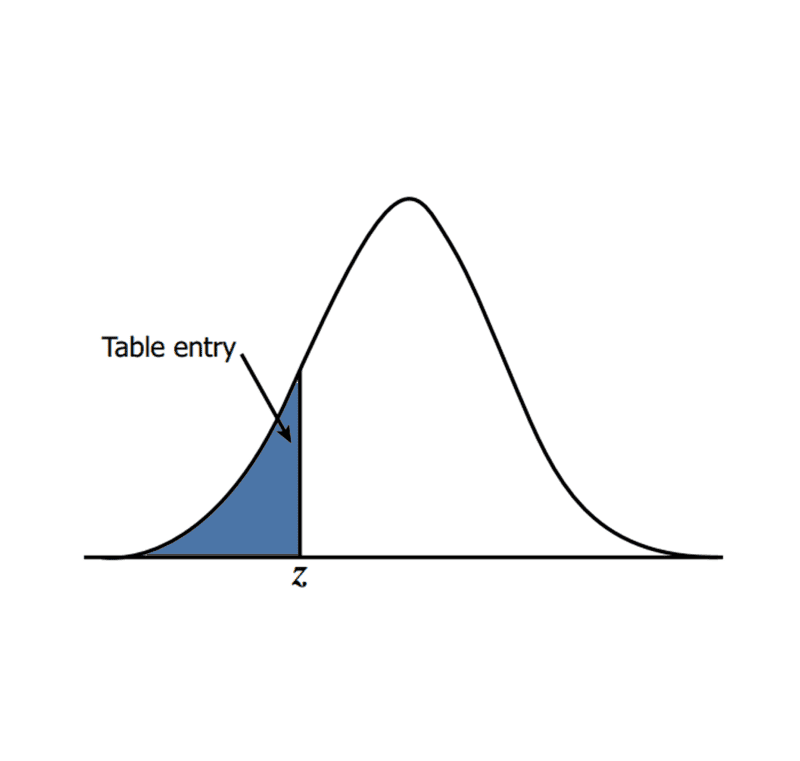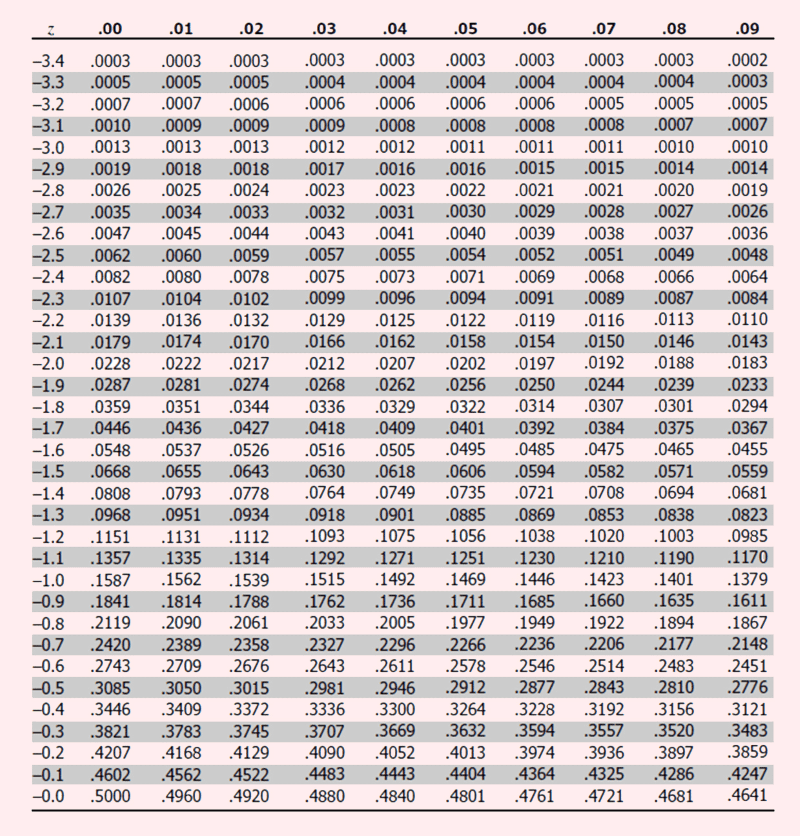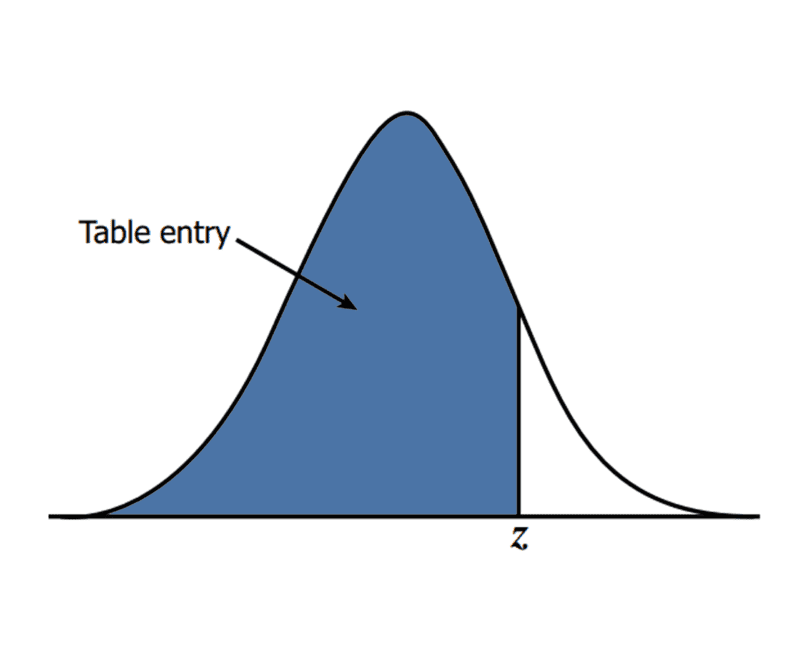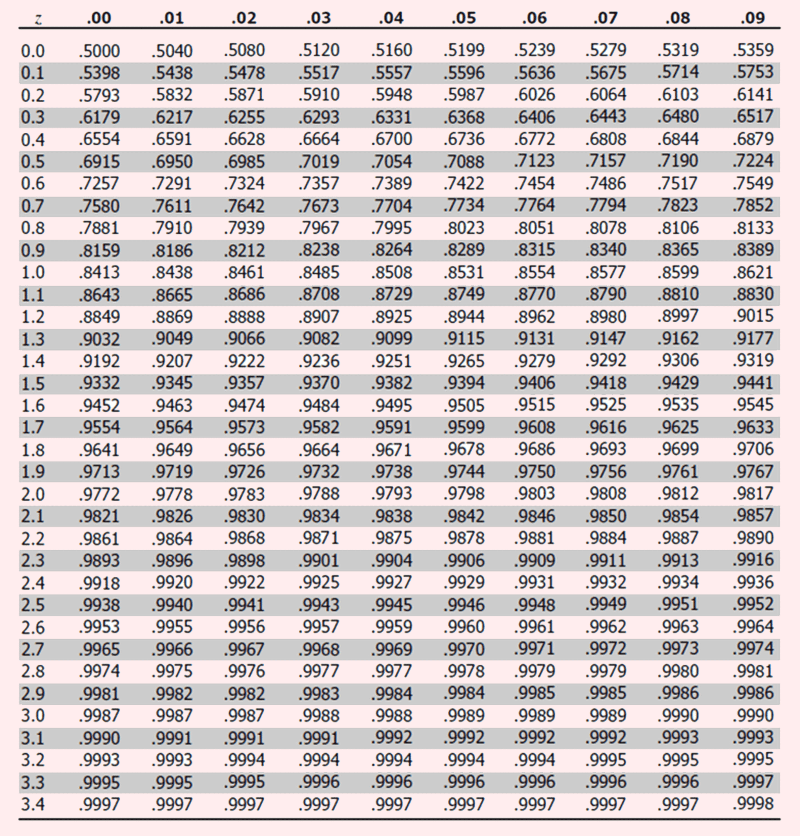Home / Blog / Data Science / Z - Table

# Z - Table

• by Mr. Sharat Chandra
• March 10, 2020
• 758

## Z Table

Z variable represents a standard Normal Random Variable with Mean = 0 and Standard Deviation = 1

Z table displays the area under the curve, which totals to 1. Practically the Z tables are calculated for a range of +/- 3.4 values (refer to six sigma concepts)

### Shows the percent of population:

• Less than or equal to Z (option "Up to Z")
• Greater than Z (option "Z onward") is calculated as 1 - (less than or equal to Z)### Example 1: Learn how to use the Z table for calculating the probability of a Continuous Random Variable

Assume a continuous random variable students examination scores following normal distribution, with µ = 711, σ = 29

Find out the probability of students securing a score of x ≤ 680?

Ans: Standard normal variable, z value calculated for corresponding continuous random variable x (680)

Step 1: Calculate z score corresponding to 680

formula: z = (x - µ) / σ

z = (680-711)/29

z = -1.06Step 2: Refer to z table:

Calculate the probabilities using z table

Start at the row with z = -1.0, and the second decimal value is read along column 06.

The value in the z table at a z value of -1.06 is 0.1446

This implies the area under the curve (towards the left tail) from -1.06 of z value is around 14.46%.

This can be read as the students' population falling between 0 and 680 scores has a probability of 14.46% for the given normal distributed data.Example 2: Assume a continuous random variable students examination scores following a normal distribution, with µ = 711, σ = 29

Find out the probability of students securing a score of x > 725?

Ans: Standard normal variable, z value calculated for corresponding continuous random variable x (725)

Step 1: Calculate z score corresponding to 725

formula: z = (x - µ) / σ

z = (725 - 711)/29

z = 0.48Step 2: Refer to z table:

Calculate the probabilities using z table

Start at the row with z = 0.4, and the second decimal value is read along column 08.

The value in the z table at the z value of 0.48 is 0.6844

This implies the area under the curve (towards the left tail) from 0.48 of z value is around 68.44%.

This can be read as the students' population falling between 0 to 725 scores has a probability of 68.44%.

The table also called the Standard Normal Table, represents the area under the curve (probability), from a given quartile value (z value) towards the left tail of the curve.

Click here to learn Data Science Course, Data Science Course in Hyderabad, Data Science Course in Bangalore

### You may also like...Interview Questions
August 06, 2020

Machine learning is a subset of data science which focuses mostly on building models by using the data.The models are built in asunch as way where they can learn themselves and improve efficiency,Interview Questions
August 05, 2020

What are the various mathematical operations within Python? Can you explain the Python code used to perform these operations?Interview Questions
July 30, 2020

Machine learning is a subset of data science which focuses mostly on building models by using the data.The models are built in asunch as way where they can learn themselves and improve efficiency,